## given that the mass density of the gold is roughly 1.91 x 10^4 kg/m^3

Question

given that the mass density of the gold is roughly 1.91 x 10^4 kg/m^3

in progress 0
4 weeks 2021-08-17T16:08:27+00:00 1 Answers 0 views 0

1. Given that,

Mass density of gold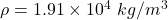Suppose, The volume of gold is 1.23 cm³

We need to calculate the mass of gold

Using formula of density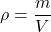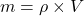Where, m = mass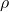=density of gold

V = volume

Put the value into the formula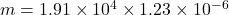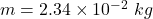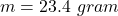Hence, The mass of gold is 23.4 gram.